# Selina Solutions Concise Maths Class 7 Chapter 19: Congruency: Congruent Triangles

Selina Solutions Concise Maths Class 7 Chapter 19 Congruency: Congruent Triangles provides students with a clear idea about the fundamentals of congruency. The solutions contain stepwise explanations in simple language to boost interest among students towards the subject. The answers are prepared in an interactive manner, which covers all the important shortcut tricks in solving problems in less time. Selina Solutions Concise Maths Class 7 Chapter 19 Congruency: Congruent Triangles, PDF links are given here with a free download option.

Chapter 19 contains important topics like congruency in triangles, corresponding sides and angles and the conditions of congruency. The solutions PDF help students clear their doubts by themselves and solve tricky problems with ease.

## Selina Solutions Concise Maths Class 7 Chapter 19: Congruency: Congruent Triangles Download PDF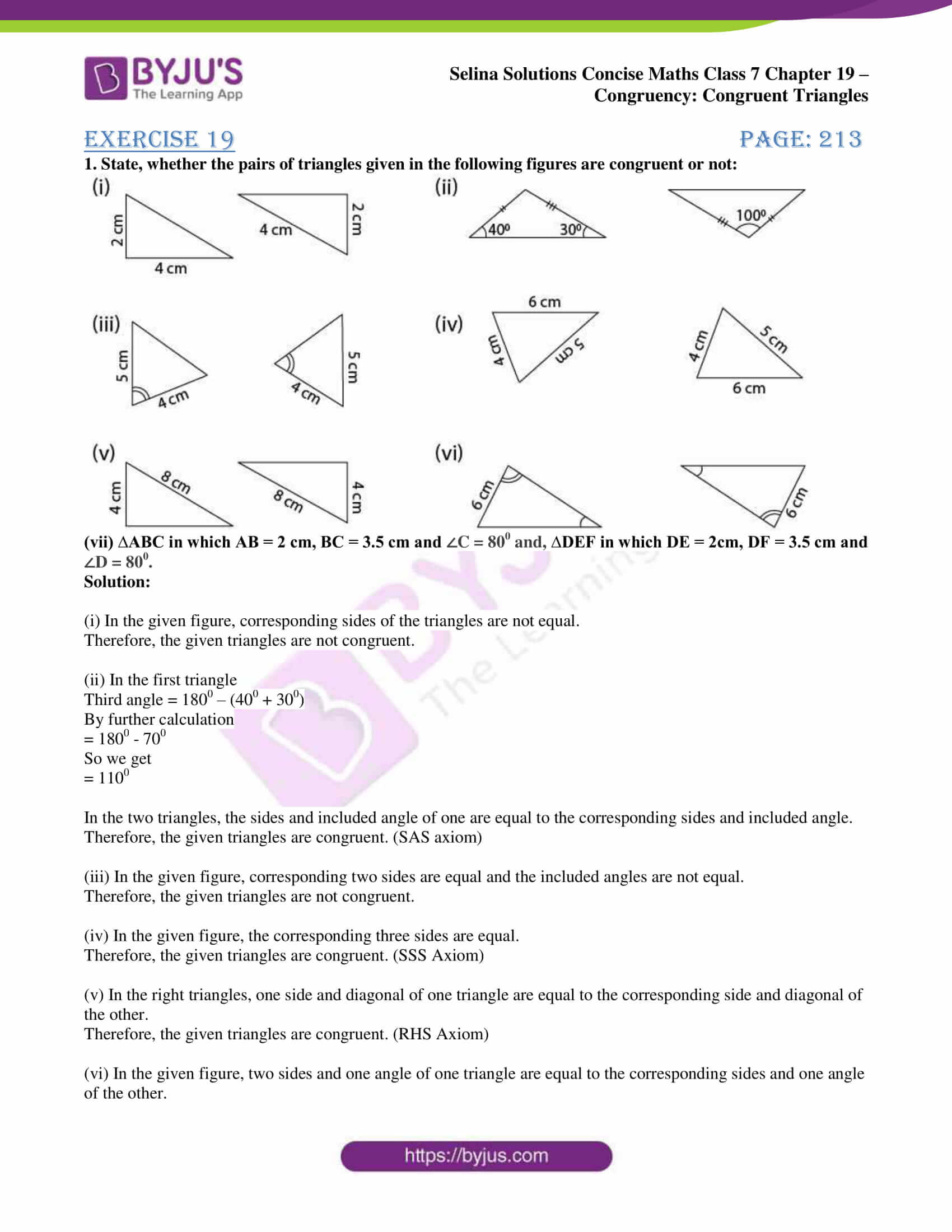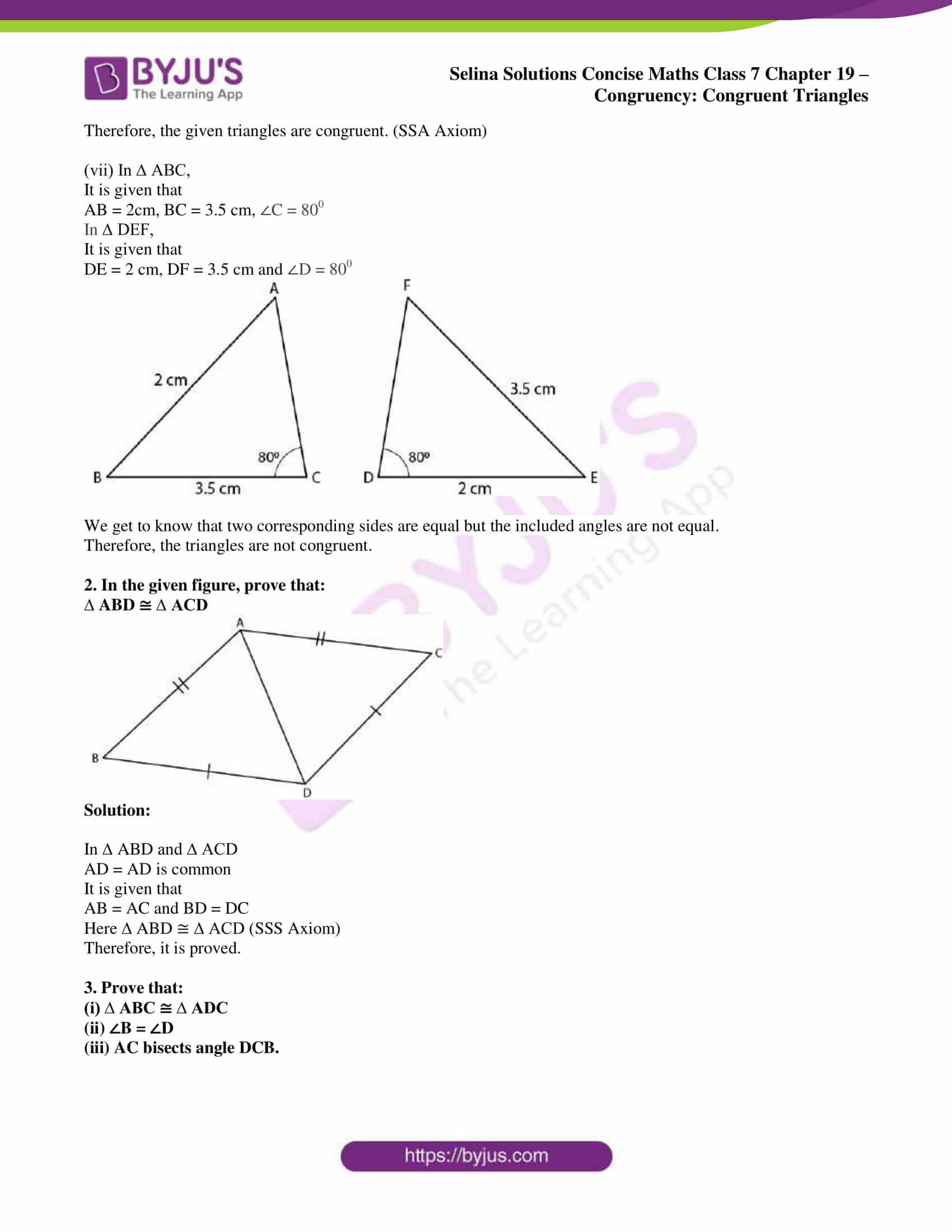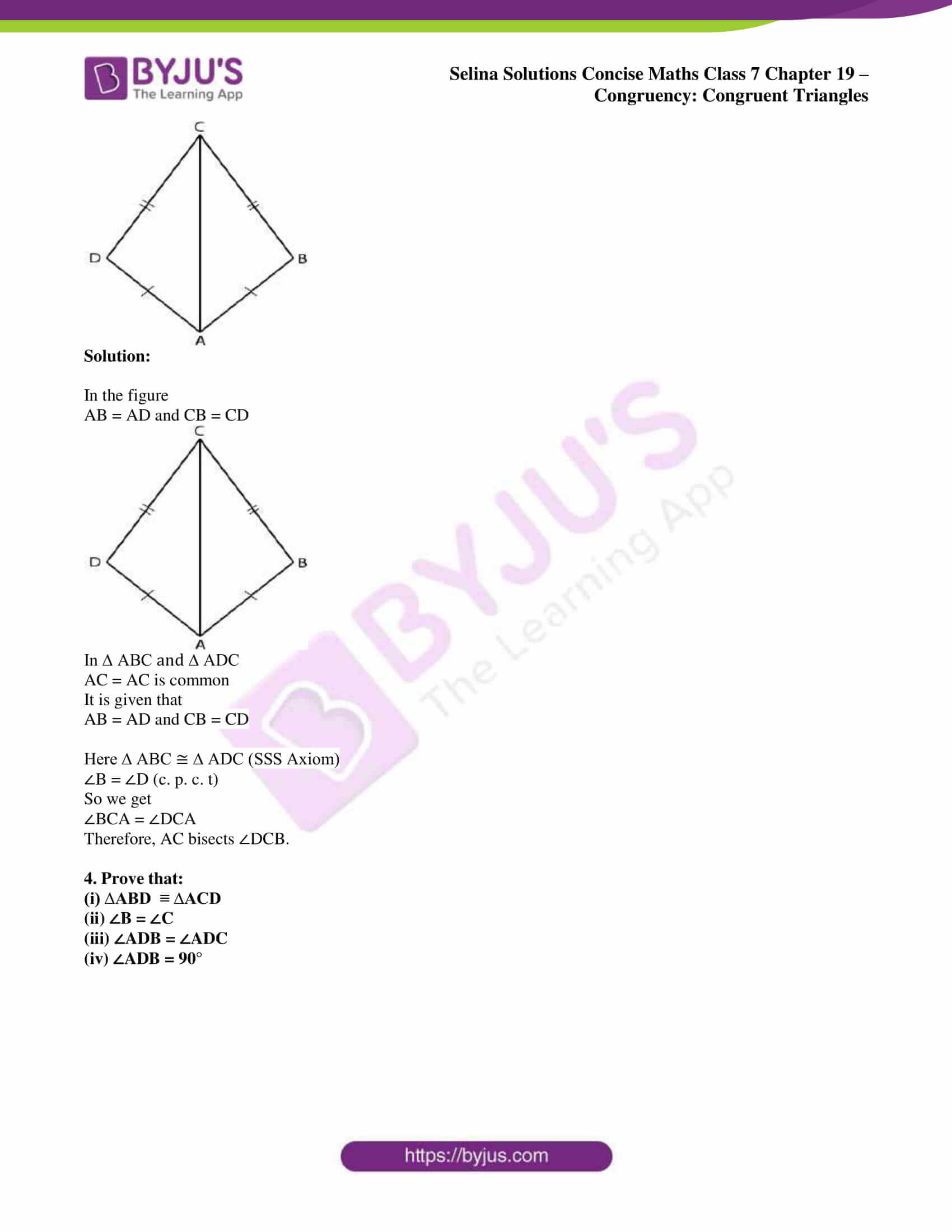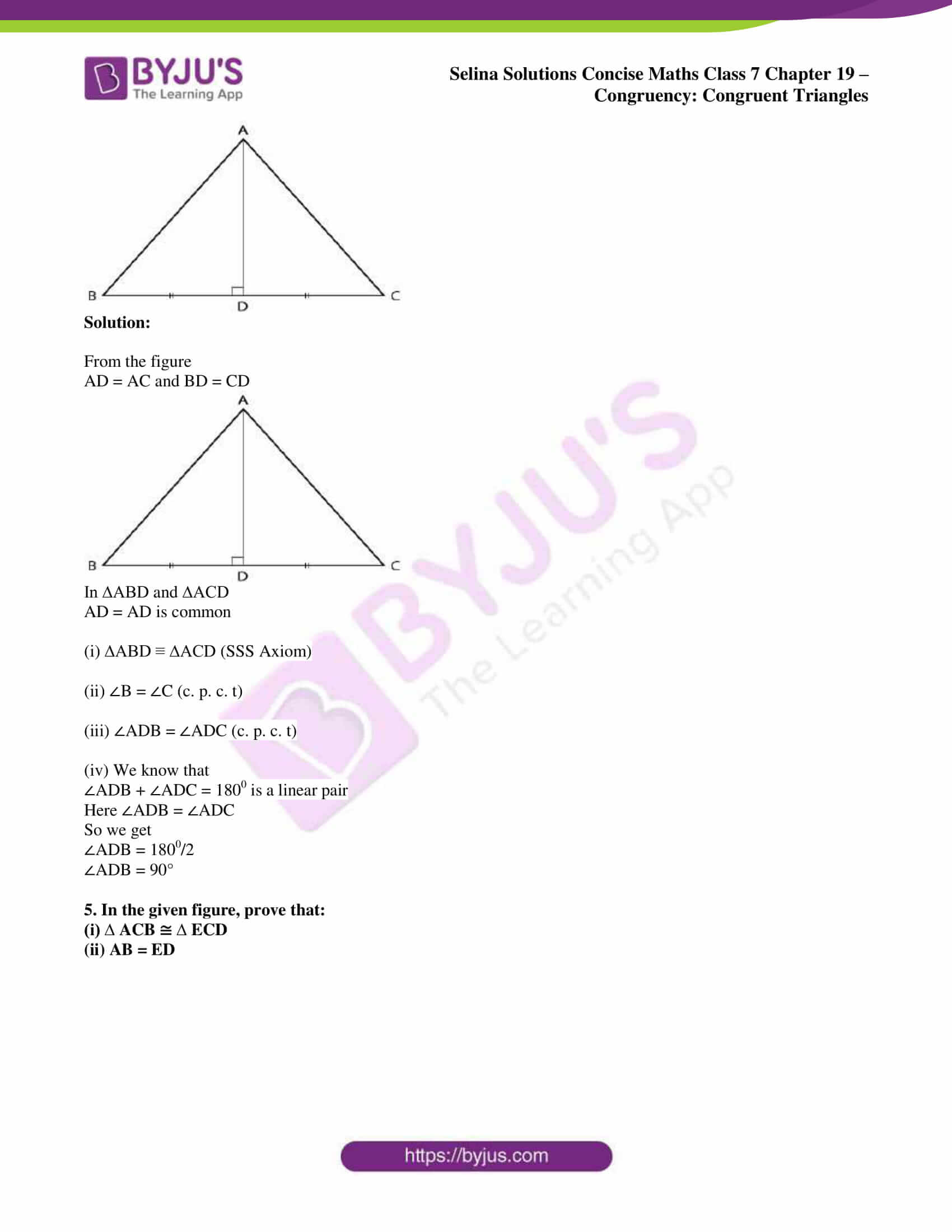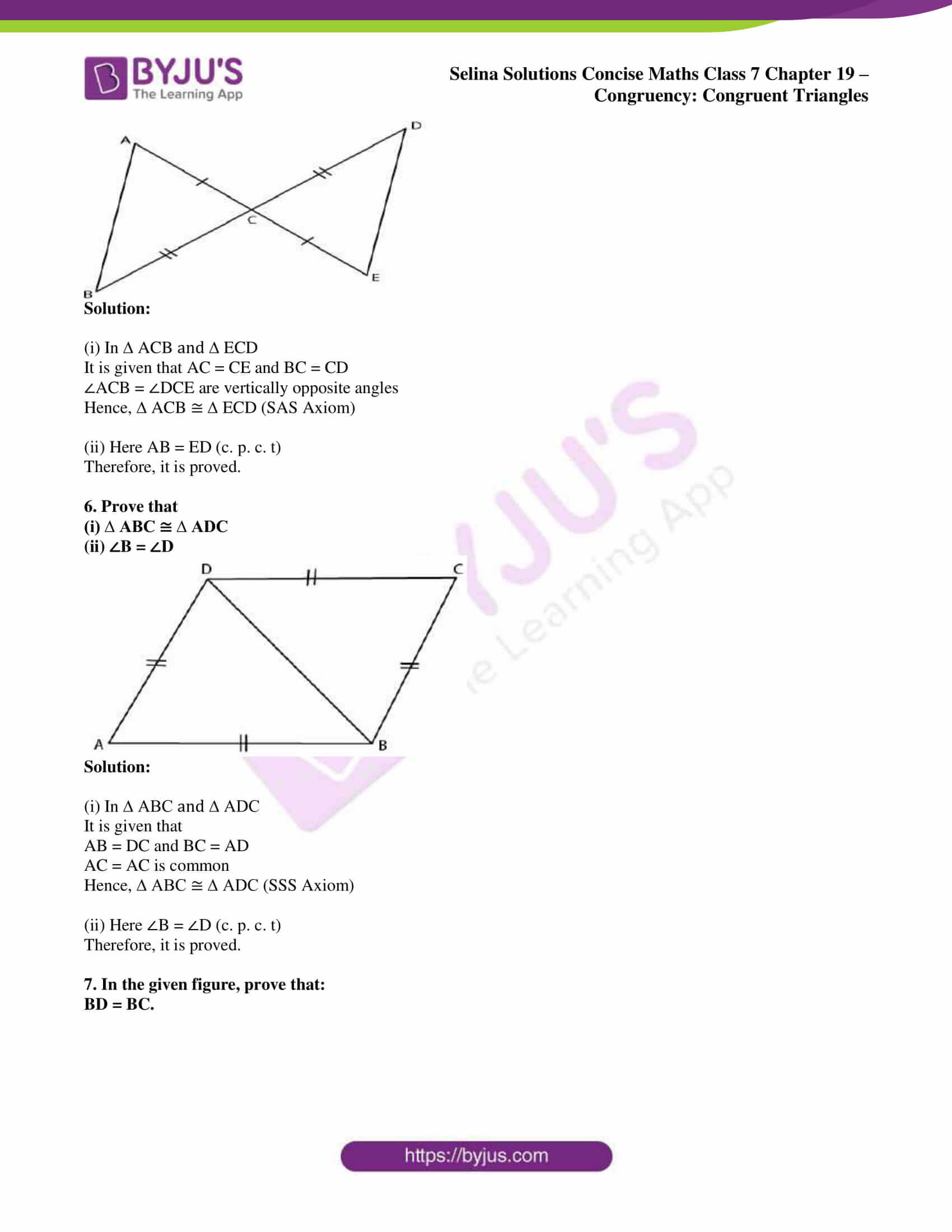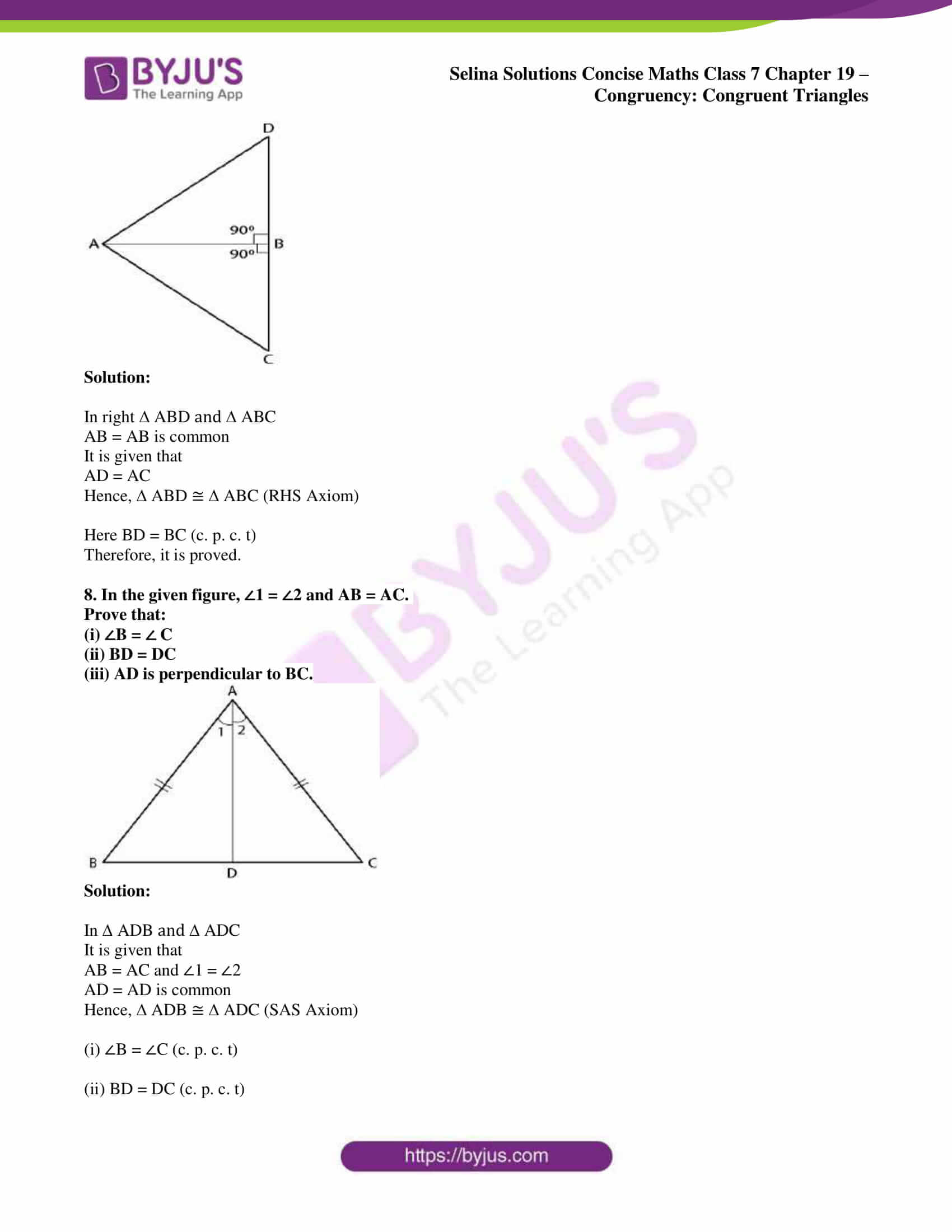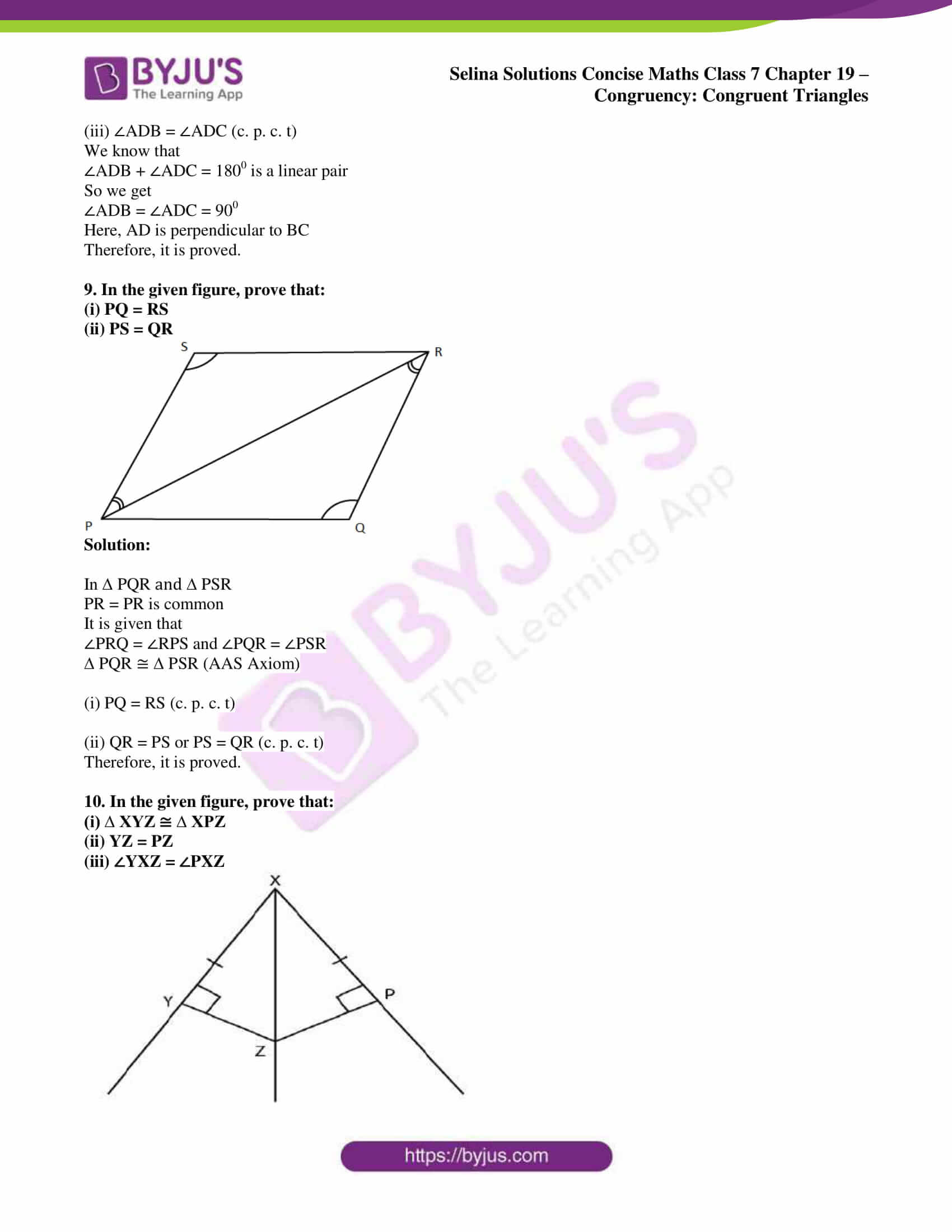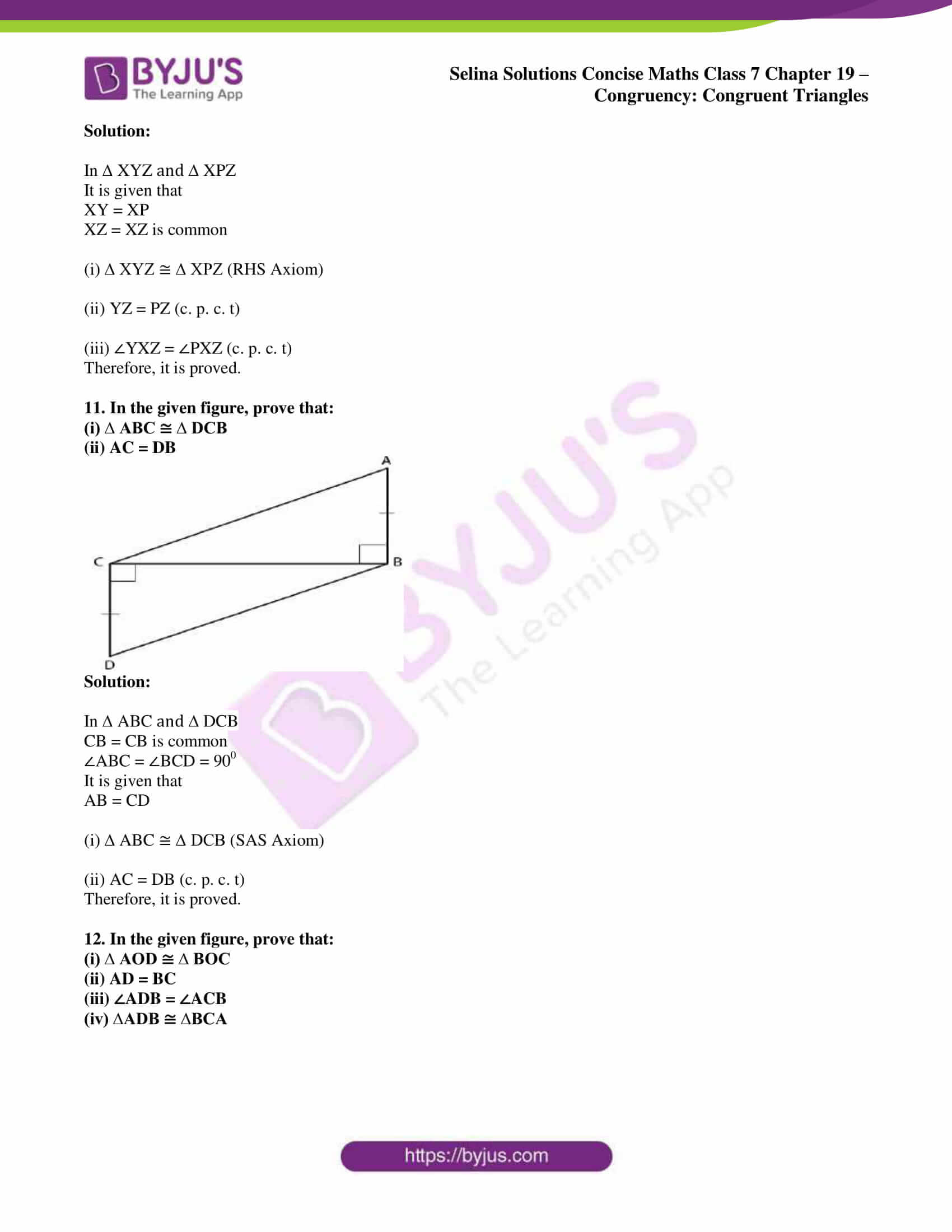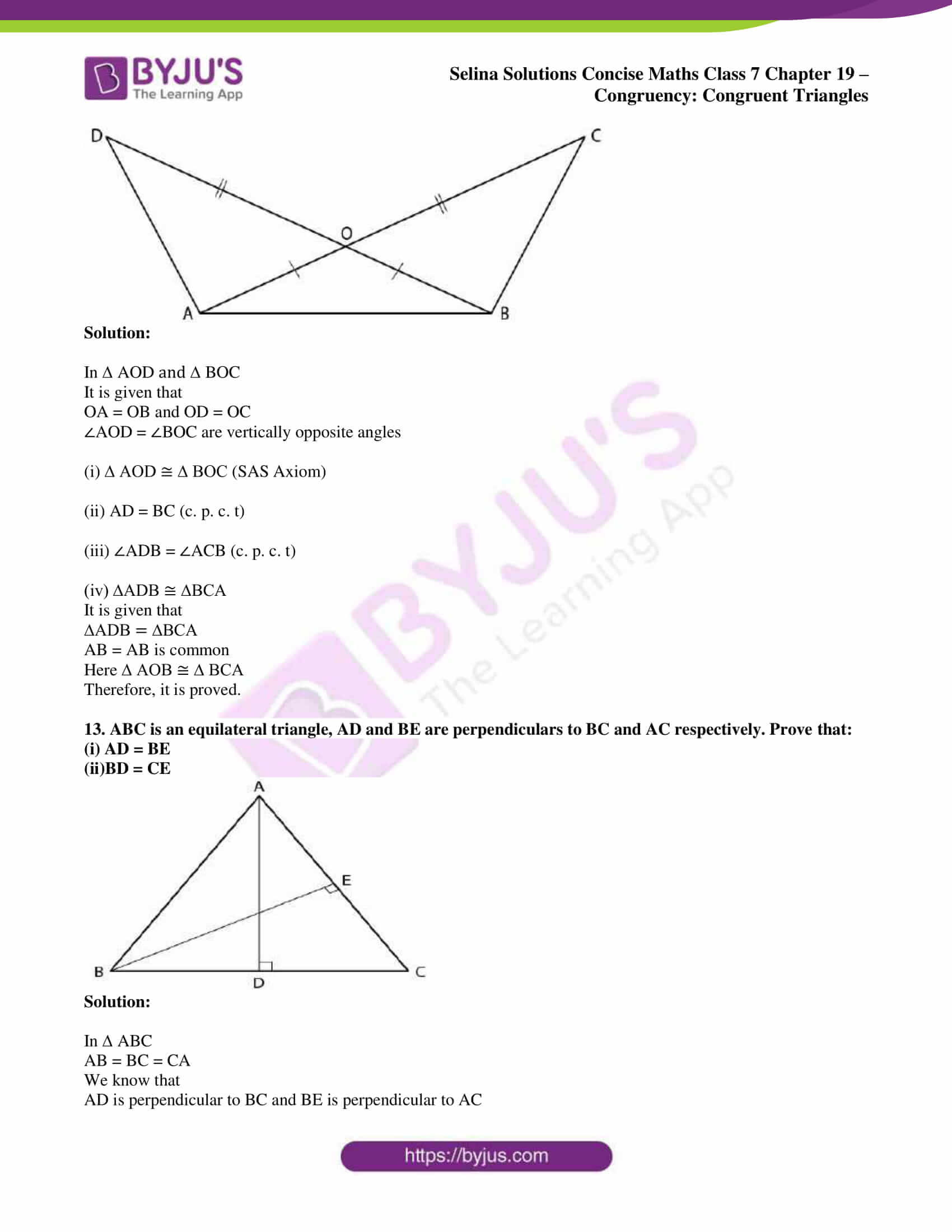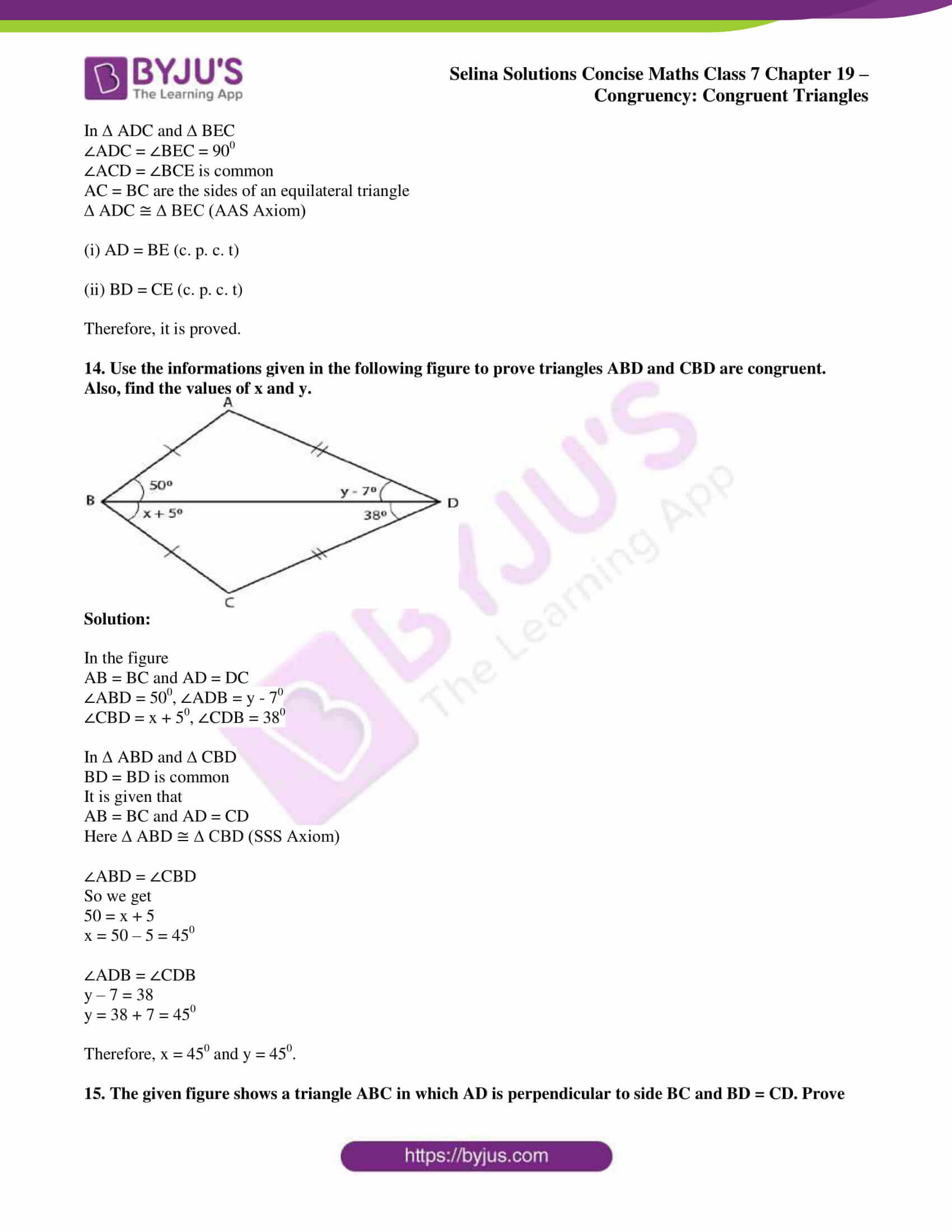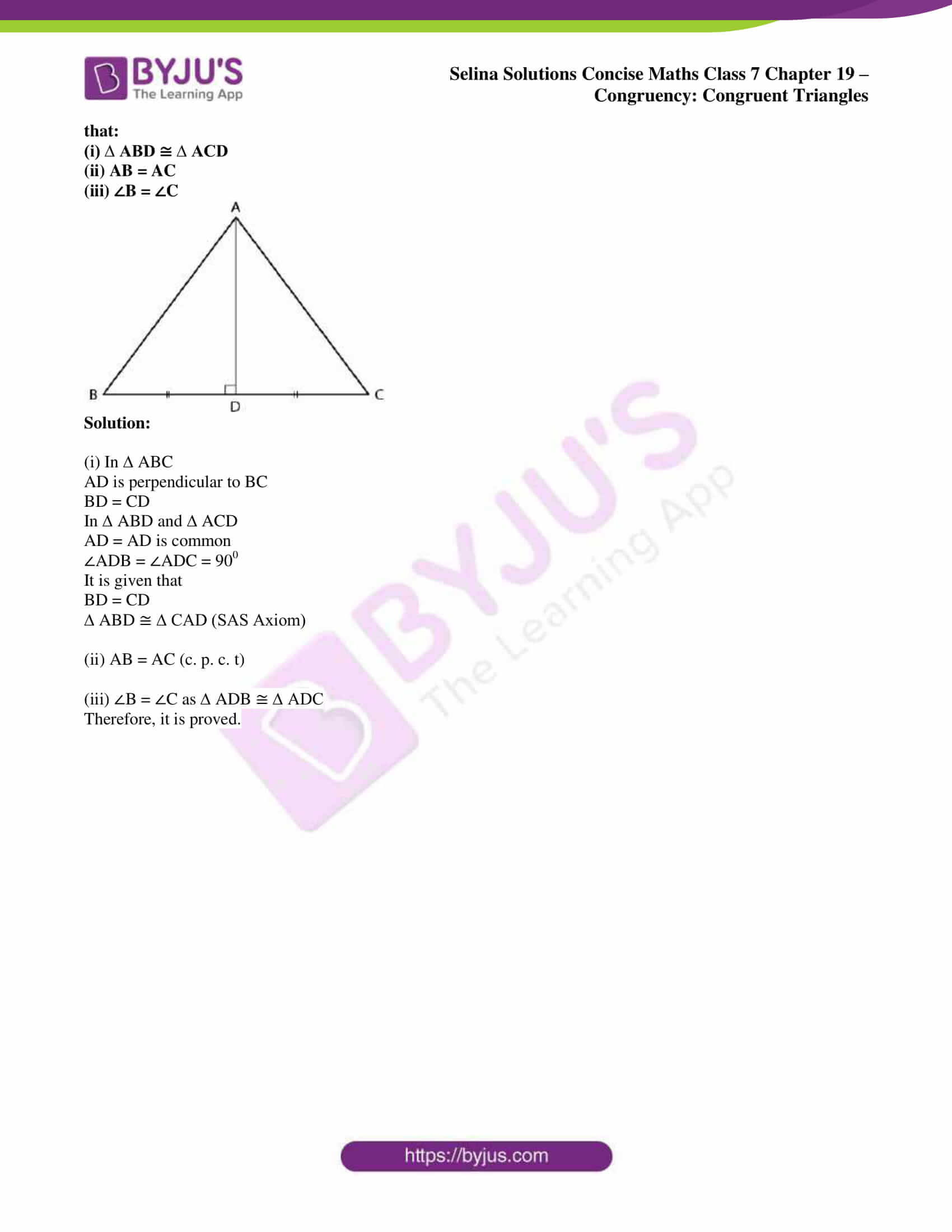## Access Selina Solutions Concise Maths Class 7 Chapter 19: Congruency: Congruent Triangles

Exercise 19 page: 213

1. State, whether the pairs of triangles given in the following figures are congruent or not: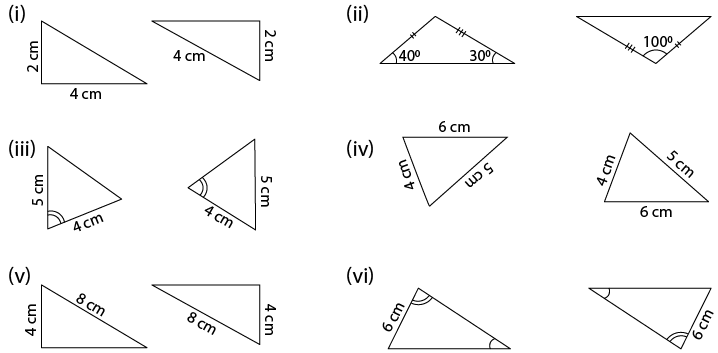(vii) ∆ABC in which AB = 2 cm, BC = 3.5 cm and ∠C = 800 and, ∆DEF in which DE = 2cm, DF = 3.5 cm and ∠D = 800.

Solution:

(i) In the given figure, corresponding sides of the triangles are not equal.

Therefore, the given triangles are not congruent.

(ii) In the first triangle

Third angle = 1800 – (400 + 300)

By further calculation

= 1800 – 700

So we get

= 1100

In the two triangles, the sides and included angle of one are equal to the corresponding sides and included angle.

Therefore, the given triangles are congruent. (SAS axiom)

(iii) In the given figure, corresponding two sides are equal and the included angles are not equal.

Therefore, the given triangles are not congruent.

(iv) In the given figure, the corresponding three sides are equal.

Therefore, the given triangles are congruent. (SSS Axiom)

(v) In the right triangles, one side and diagonal of one triangle are equal to the corresponding side and diagonal of the other.

Therefore, the given triangles are congruent. (RHS Axiom)

(vi) In the given figure, two sides and one angle of one triangle are equal to the corresponding sides and one angle of the other.

Therefore, the given triangles are congruent. (SSA Axiom)

(vii) In ∆ ABC,

It is given that

AB = 2cm, BC = 3.5 cm, ∠C = 800

In ∆ DEF,

It is given that

DE = 2 cm, DF = 3.5 cm and ∠D = 800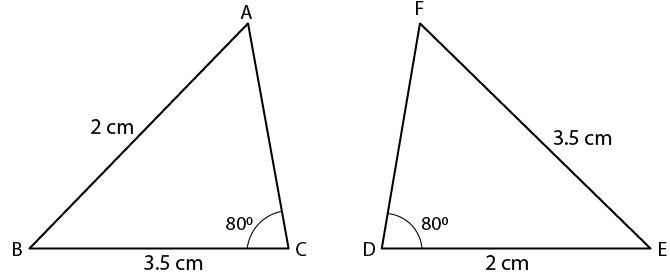We get to know that two corresponding sides are equal but the included angles are not equal.

Therefore, the triangles are not congruent.

2. In the given figure, prove that:

∆ ABD ≅ ∆ ACD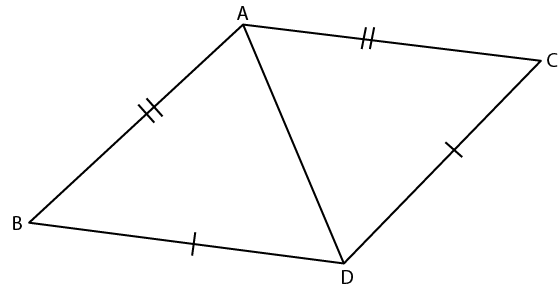Solution:

In ∆ ABD and ∆ ACD

It is given that

AB = AC and BD = DC

Here ∆ ABD ≅ ∆ ACD (SSS Axiom)

Therefore, it is proved.

3. Prove that:

(i) ∆ ABC ≅ ∆ ADC

(ii) ∠B = ∠D

(iii) AC bisects angle DCB.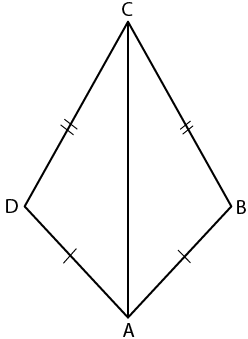Solution:

In the figure

AB = AD and CB = CDIn ∆ ABC and ∆ ADC

AC = AC is common

It is given that

AB = AD and CB = CD

Here ∆ ABC ≅ ∆ ADC (SSS Axiom)

∠B = ∠D (c. p. c. t)

So we get

∠BCA = ∠DCA

Therefore, AC bisects ∠DCB.

4. Prove that:

(i) ∆ABD  ≡ ∆ACD

(ii) ∠B = ∠C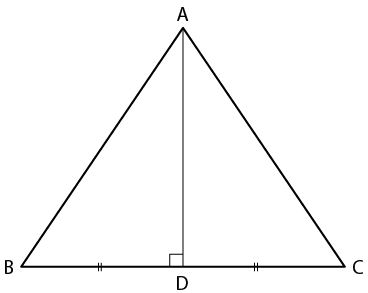Solution:

From the figure

AD = AC and BD = CDIn ∆ABD and ∆ACD

(i) ∆ABD ≡ ∆ACD (SSS Axiom)

(ii) ∠B = ∠C (c. p. c. t)

(iv) We know that

So we get

5. In the given figure, prove that:

(i) ∆ ACB ≅ ∆ ECD

(ii) AB = ED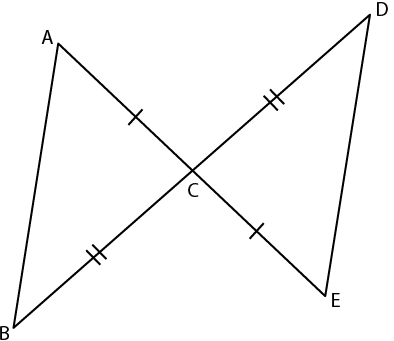Solution:

(i) In ∆ ACB and ∆ ECD
It is given that AC = CE and BC = CD

∠ACB = ∠DCE are vertically opposite angles

Hence, ∆ ACB ≅ ∆ ECD (SAS Axiom)

(ii) Here AB = ED (c. p. c. t)

Therefore, it is proved.

6. Prove that

(i) ∆ ABC ≅ ∆ ADC

(ii) ∠B = ∠D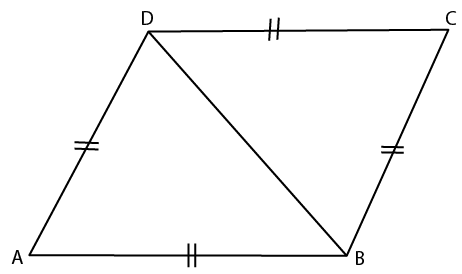Solution:

(i) In ∆ ABC and ∆ ADC

It is given that

AB = DC and BC = AD

AC = AC is common

Hence, ∆ ABC ≅ ∆ ADC (SSS Axiom)

(ii) Here ∠B = ∠D (c. p. c. t)

Therefore, it is proved.

7. In the given figure, prove that:

BD = BC.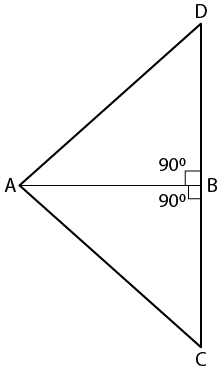Solution:

In right ∆ ABD and ∆ ABC

AB = AB is common

It is given that

Hence, ∆ ABD ≅ ∆ ABC (RHS Axiom)

Here BD = BC (c. p. c. t)

Therefore, it is proved.

8. In the given figure, ∠1 = ∠2 and AB = AC.

Prove that:
(i) ∠B = ∠ C
(ii) BD = DC
(iii) AD is perpendicular to BC.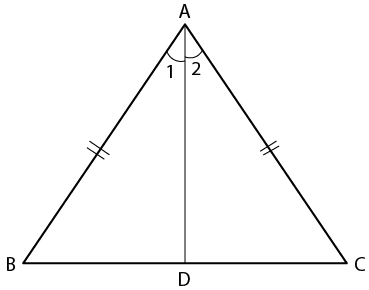Solution:

It is given that

AB = AC and ∠1 = ∠2

(i) ∠B = ∠C (c. p. c. t)

(ii) BD = DC (c. p. c. t)

We know that

So we get

Here, AD is perpendicular to BC

Therefore, it is proved.

9. In the given figure, prove that:
(i) PQ = RS
(ii) PS = QR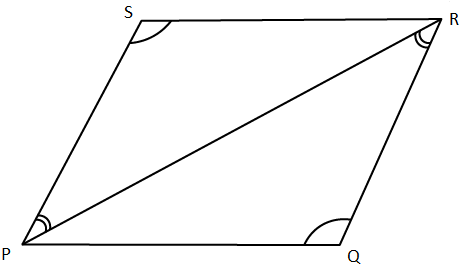Solution:

In ∆ PQR and ∆ PSR

PR = PR is common

It is given that

∠PRQ = ∠RPS and ∠PQR = ∠PSR

∆ PQR ≅ ∆ PSR (AAS Axiom)

(i) PQ = RS (c. p. c. t)

(ii) QR = PS or PS = QR (c. p. c. t)

Therefore, it is proved.

10. In the given figure, prove that:

(i) ∆ XYZ ≅ ∆ XPZ
(ii) YZ = PZ
(iii) ∠YXZ = ∠PXZ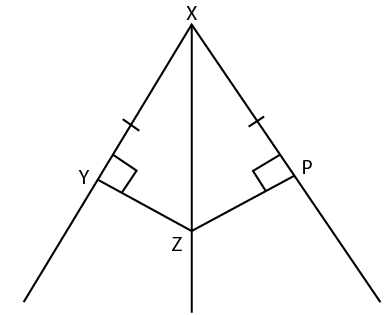Solution:

In ∆ XYZ and ∆ XPZ

It is given that

XY = XP

XZ = XZ is common

(i) ∆ XYZ ≅ ∆ XPZ (RHS Axiom)

(ii) YZ = PZ (c. p. c. t)

(iii) ∠YXZ = ∠PXZ (c. p. c. t)

Therefore, it is proved.

11. In the given figure, prove that:

(i) ∆ ABC ≅ ∆ DCB

(ii) AC = DB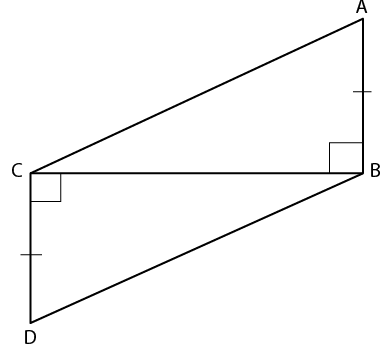Solution:

In ∆ ABC and ∆ DCB

CB = CB is common

∠ABC = ∠BCD = 900

It is given that

AB = CD

(i) ∆ ABC ≅ ∆ DCB (SAS Axiom)

(ii) AC = DB (c. p. c. t)

Therefore, it is proved.

12. In the given figure, prove that:

(i) ∆ AOD ≅ ∆ BOC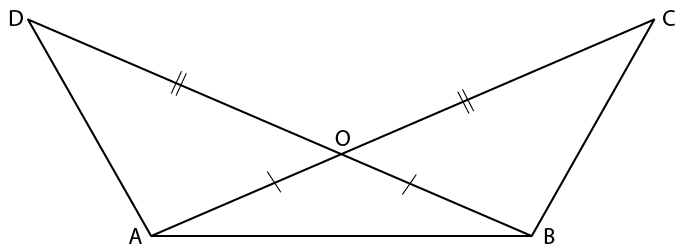Solution:

In ∆ AOD and ∆ BOC

It is given that

OA = OB and OD = OC

∠AOD = ∠BOC are vertically opposite angles

(i) ∆ AOD ≅ ∆ BOC (SAS Axiom)

(ii) AD = BC (c. p. c. t)

(iii) ∠ADB = ∠ACB (c. p. c. t)

It is given that

AB = AB is common

Here ∆ AOB ≅ ∆ BCA

Therefore, it is proved.

13. ABC is an equilateral triangle, AD and BE are perpendiculars to BC and AC respectively. Prove that:
(ii)BD = CE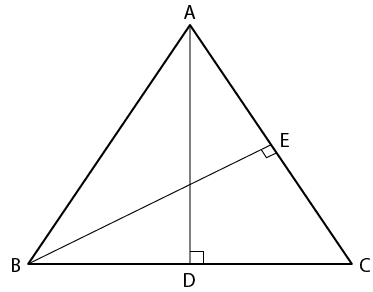Solution:

In ∆ ABC

AB = BC = CA

We know that

AD is perpendicular to BC and BE is perpendicular to AC

In ∆ ADC and ∆ BEC

∠ACD = ∠BCE is common

AC = BC are the sides of an equilateral triangle

∆ ADC ≅ ∆ BEC (AAS Axiom)

(i) AD = BE (c. p. c. t)

(ii) BD = CE (c. p. c. t)

Therefore, it is proved.

14. Use the informations given in the following figure to prove triangles ABD and CBD are congruent.

Also, find the values of x and y.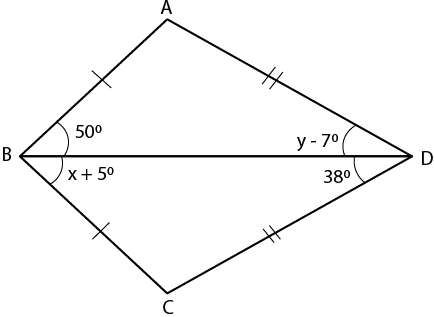Solution:

In the figure

AB = BC and AD = DC

∠ABD = 500, ∠ADB = y – 70

∠CBD = x + 50, ∠CDB = 380

In ∆ ABD and ∆ CBD

BD = BD is common

It is given that

AB = BC and AD = CD

Here ∆ ABD ≅ ∆ CBD (SSS Axiom)

∠ABD = ∠CBD

So we get

50 = x + 5

x = 50 – 5 = 450

y – 7 = 38

y = 38 + 7 = 450

Therefore, x = 450 and y = 450.

15. The given figure shows a triangle ABC in which AD is perpendicular to side BC and BD = CD. Prove that:
(i) ∆ ABD ≅ ∆ ACD
(ii) AB = AC
(iii) ∠B = ∠C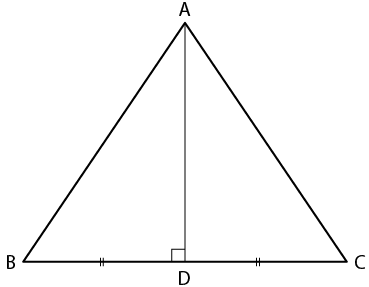Solution:

(i) In ∆ ABC

BD = CD

In ∆ ABD and ∆ ACD